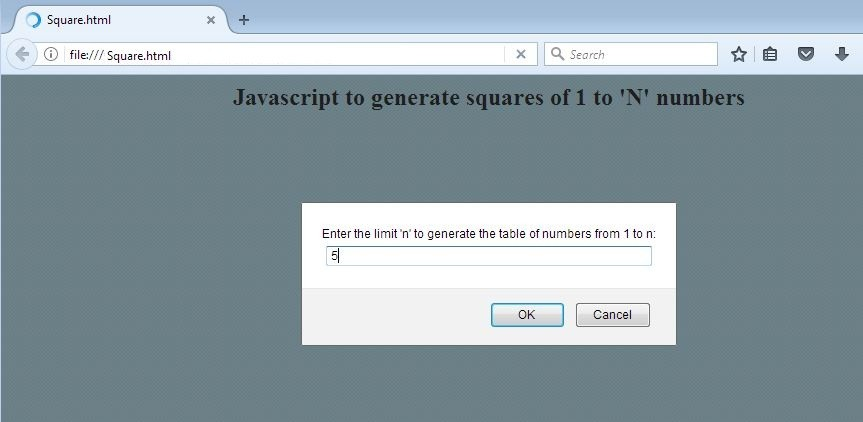# Javascript to generate squares of 1 to n numbers

This post contains the Javascript to generate squares of 1 to n numbers. This script accepts a number as the input and generates the squares of all ‘n’ numbers starting from 1. Please go through the video and images to understand how it works.

Input: A number n obtained using prompt
Output: A table of numbers from 1 to n and their squares

```//Square.html

<!DOCTYPE HTML>
<?xml version="1.0" encoding="utf-8"?>
<html xmlns="http://www.w3.org/1999/xhtml">

<title> Square.html </title>
<script type="text/javascript">
function square()
{
var n=prompt ("Enter the limit 'n' to generate the table of numbers from 1 to n:","");
var msg="";
var res= "0";

for(var x= 1; x<=n;x++)
{
res = x * x;
msg = msg + " " + x + " * "+ x + " = " + res + "\n";
}

}
</script>

<body style = "background-color:lightblue" onload = "square();">
<h2 style="text-align:center;color:black"> Javascript to generate squares of 1 to 'N' numbers</h2>
</body>
</html>```

Video explains the execution of the javascript:

Input – a screenshot of the browser.Output showing squares for 1 to 5.Output 2 – shows squares of 1 to 15How to execute this script?

1. Copy the code and save it in a text file with a .html extension.
2. Open the same file through the browser.
3. You will be asked to enter a number and result will be displayed.
4. To check for another number, refresh the page and again enter a new number.